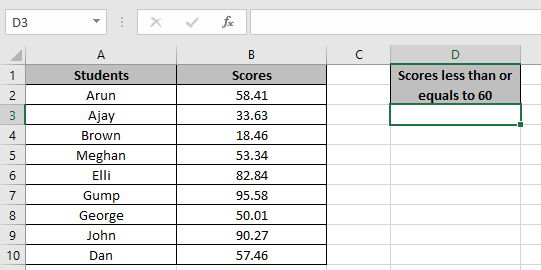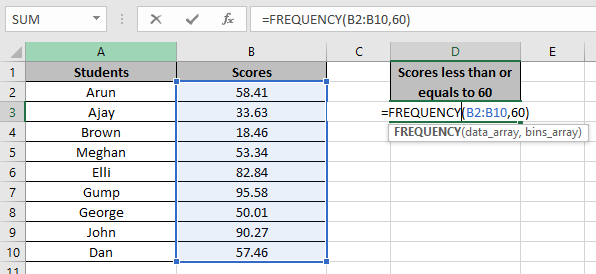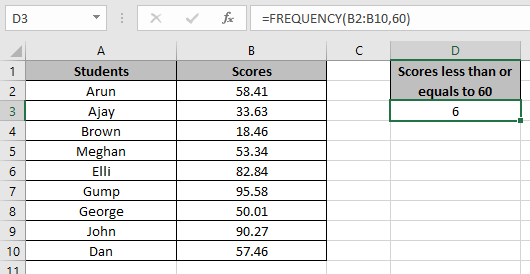# How to Use Frequency Function in Excel

Frequency function returns the count of cells from the data_array where the number is less than or equals to the number provided in bins_array.
Syntax:

=FREQUENCY( data_array, bins_array)

data_array: array of values to count
Bins_arrray: a value to check
Let’s count some cells using the function

Here we have some scores of students and we need to find the count of students who have scored less than 60.Use the formula in D3 cell

=FREQUENCY(B2:B10,60)

B2:B10: data_array
60: Value to checkPress Enter and copy the formula in other cells using the Ctrl + D shortcut key.As you can see there are 6 scores less than 60 in the array. You can customize the formula for different purposes.

Hope you understood how to use excel FREQUENCY function. Explore more articles on Excel functions here. Please state your queries in the comment box below.

Terms and Conditions of use

The applications/code on this site are distributed as is and without warranties or liability. In no event shall the owner of the copyrights, or the authors of the applications/code be liable for any loss of profit, any problems or any damage resulting from the use or evaluation of the applications/code.